Matter Matter Anything that has mass and takes

• Slides: 24Matter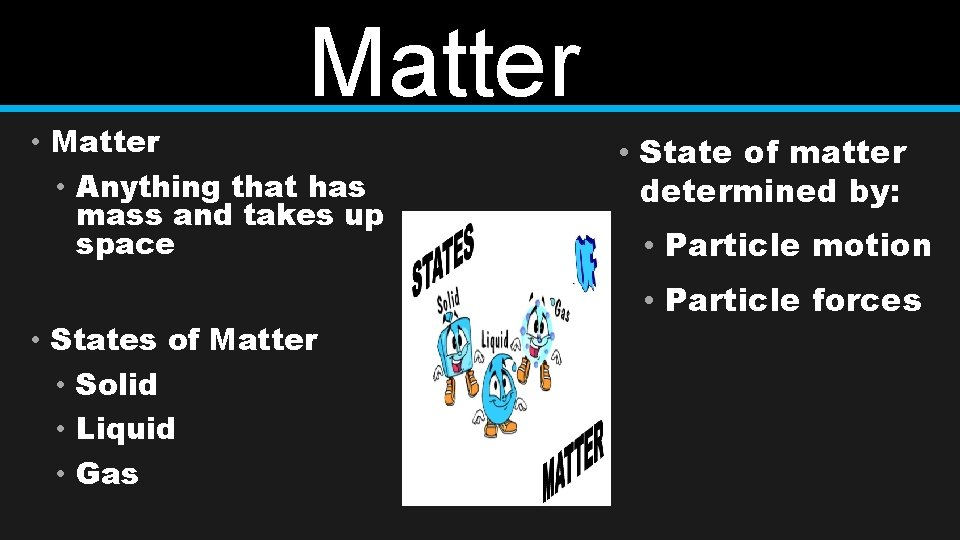Matter • Anything that has mass and takes up space • States of Matter • Solid • Liquid • Gas • State of matter determined by: • Particle motion • Particle forces• Matter that has a definite shape and a definite volume • Strong attractive forces • Slow motion • Vibrate back and forth • Particles are close together Solid • Two Types of Solids • Crystalline • Particles arranged in a specific repeating order • Amorphous • Particles arranged randomlyLiquid • Matter with a definite volume but no definite shape • Weaker attractive force • Faster moving particles • Particles spread apart • Flows and takes the shape of containers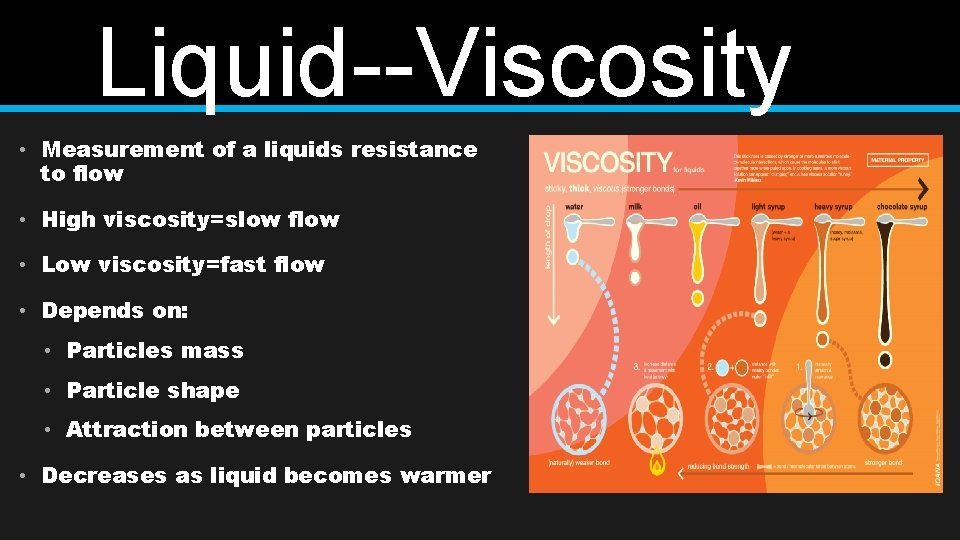Liquid--Viscosity • Measurement of a liquids resistance to flow • High viscosity=slow flow • Low viscosity=fast flow • Depends on: • Particles mass • Particle shape • Attraction between particles • Decreases as liquid becomes warmer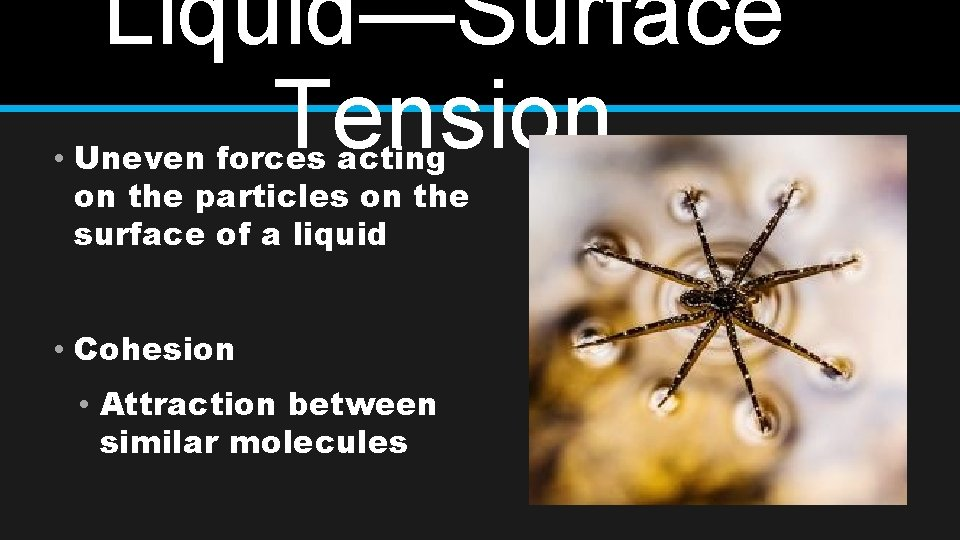Liquid—Surface Tension • Uneven forces acting on the particles on the surface of a liquid • Cohesion • Attraction between similar molecules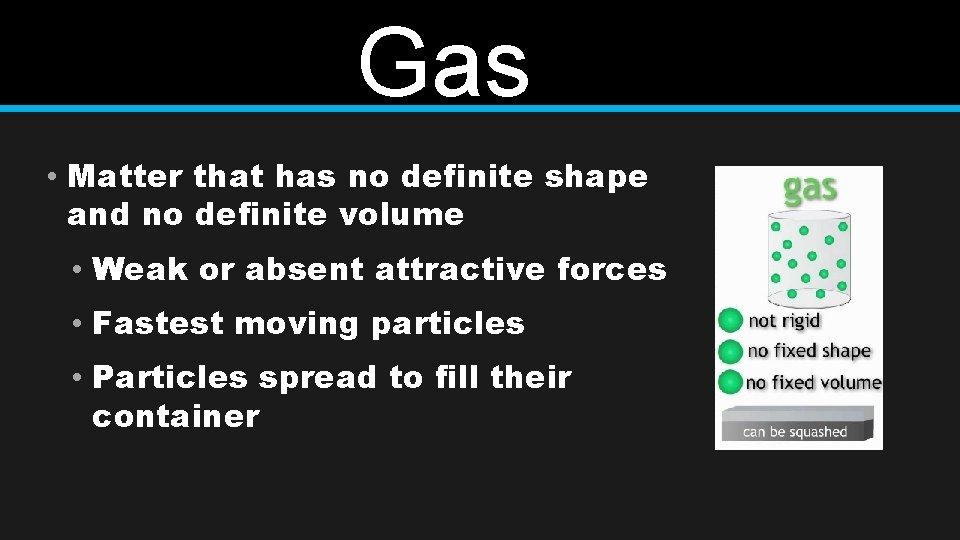Gas • Matter that has no definite shape and no definite volume • Weak or absent attractive forces • Fastest moving particles • Particles spread to fill their containerVapor • Normally a solid or liquid at room temperature • Examples: • Water, rubbing alcohol, iodine, mercury, gasoline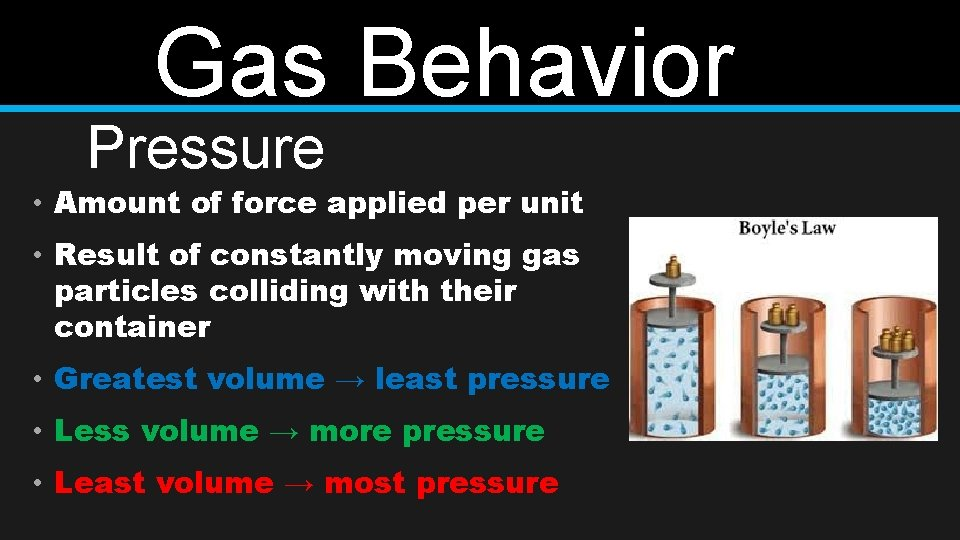Gas Behavior Pressure • Amount of force applied per unit • Result of constantly moving gas particles colliding with their container • Greatest volume → least pressure • Less volume → more pressure • Least volume → most pressure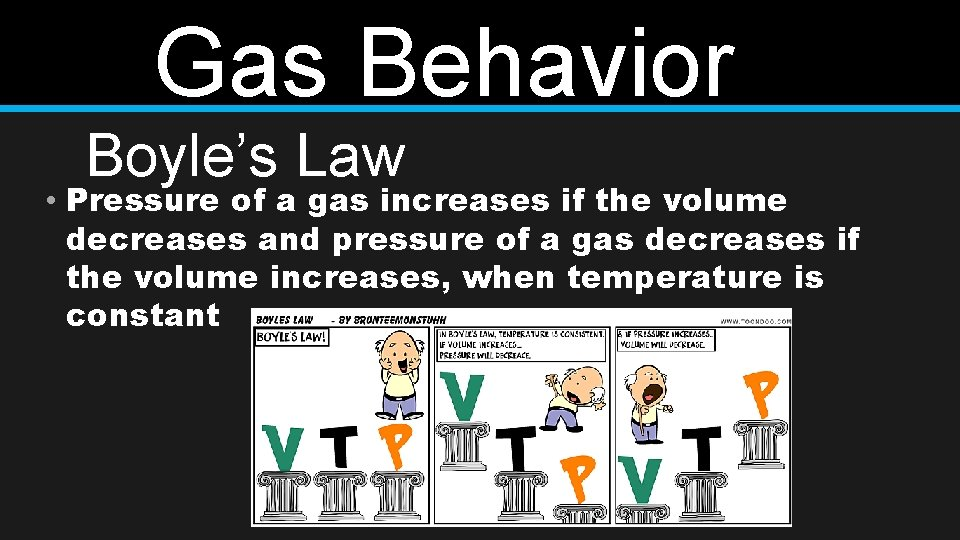Gas Behavior Boyle’s Law • Pressure of a gas increases if the volume decreases and pressure of a gas decreases if the volume increases, when temperature is constant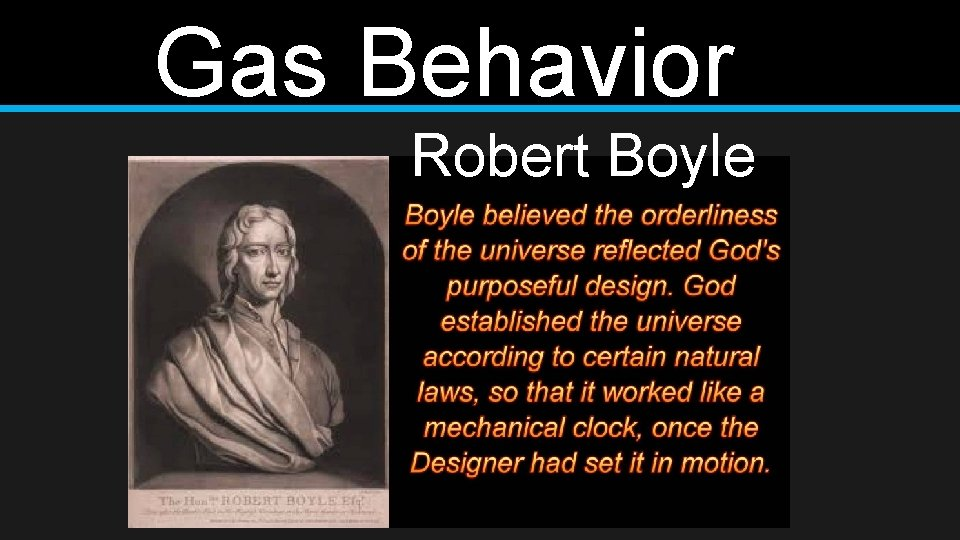Gas Behavior Robert Boyle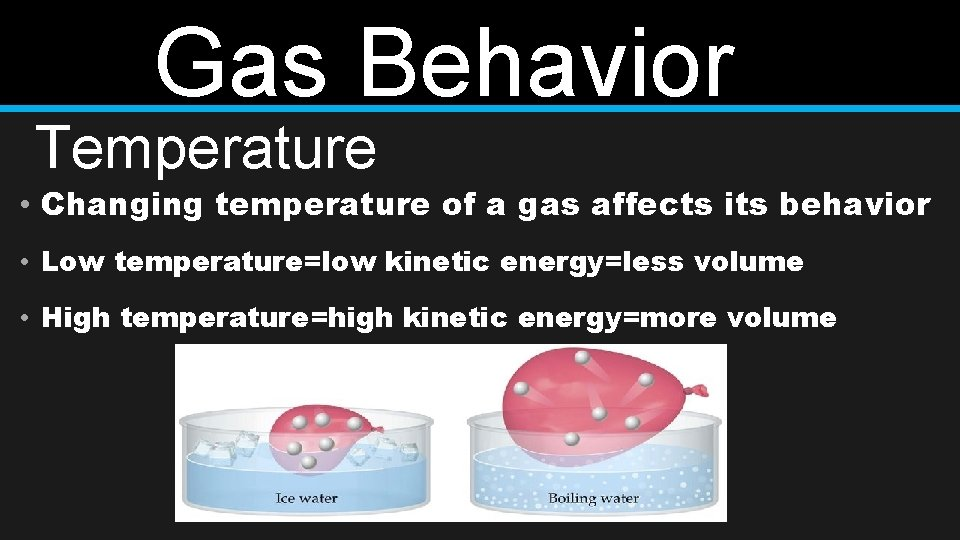Gas Behavior Temperature • Changing temperature of a gas affects its behavior • Low temperature=low kinetic energy=less volume • High temperature=high kinetic energy=more volume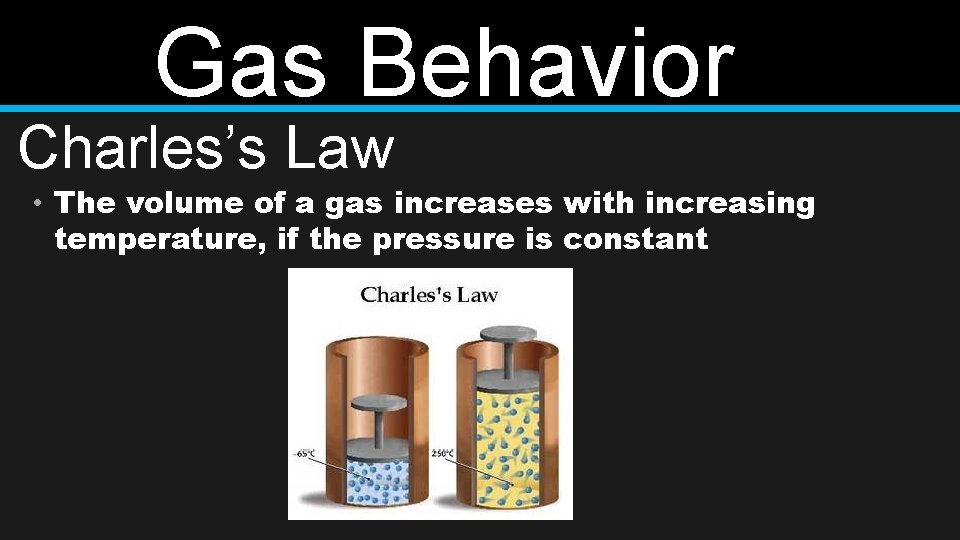Gas Behavior Charles’s Law • The volume of a gas increases with increasing temperature, if the pressure is constantGas Behavior Jacques CharlesGas Behavior Charles’s Law • The volume of a gas increases with increasing temperature, if the pressure is constantKinetic Molecular Theory • Explanation of how particles in matter behave 1. Small particles make up all matter 2. Particles are in constant, random motion 3. Particles collide with other particles, other objects, and the walls of their container 4. When particles collide, no energy is lostPhysical Properties Mass Dependent Amount of matter in an object Conductivity Independent Ability of matter to conduct electricity or heat Volume Dependent Amount of space something occupies Density Dependent Amount of mass per unit of volumePhysical Properties State of Matter Independent Solid, liquid, gas Solubility Independent Ability of one substance to dissolve in another Magnetism Independent Attractive forces for metals Boiling/ Melting Point changes state Dependent Temperature at which materialPhysical Changes • A change in size, shape, form or state of matter • Matter’s identity stays the same • Types • Change in shape or size • Change in state of matter • Adding thermal energy • Removing thermal energy • Dissolving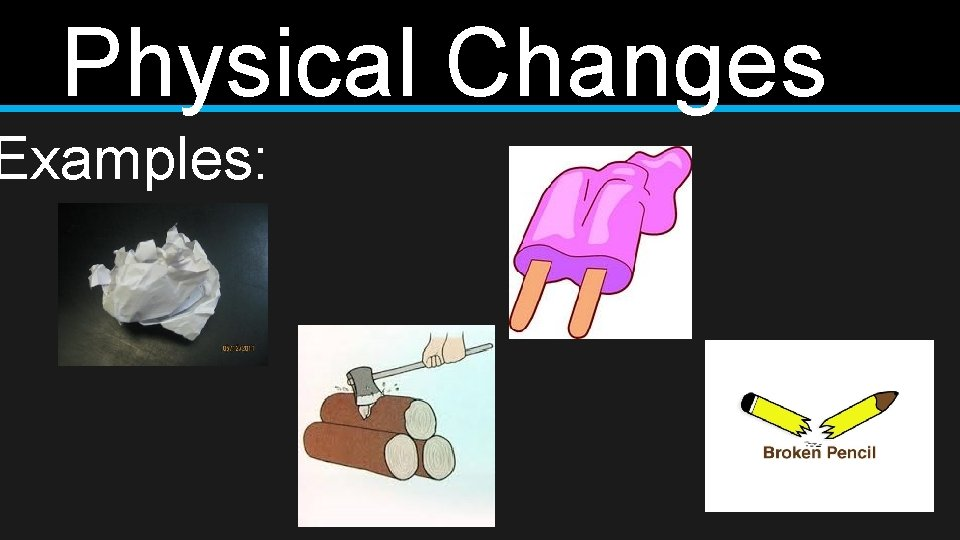Physical Changes Examples: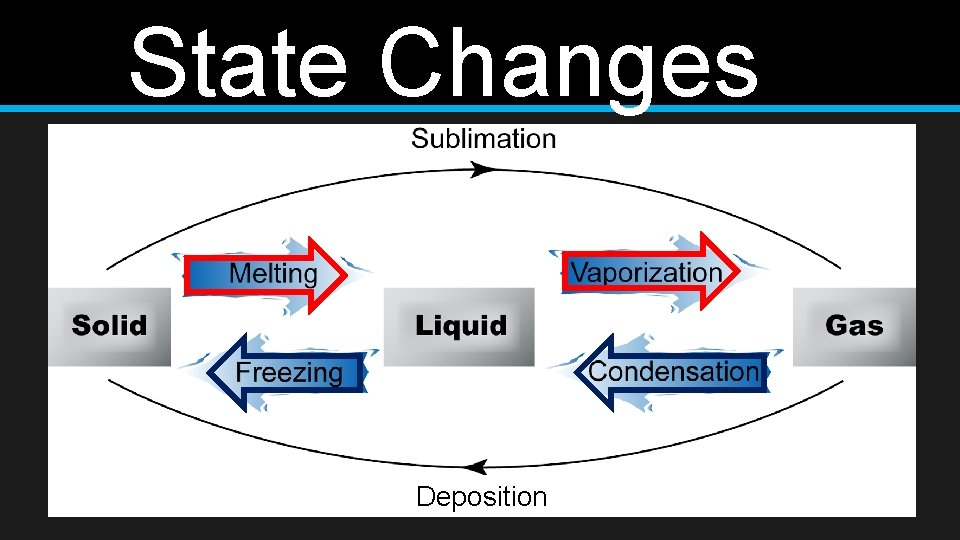State Changes Deposition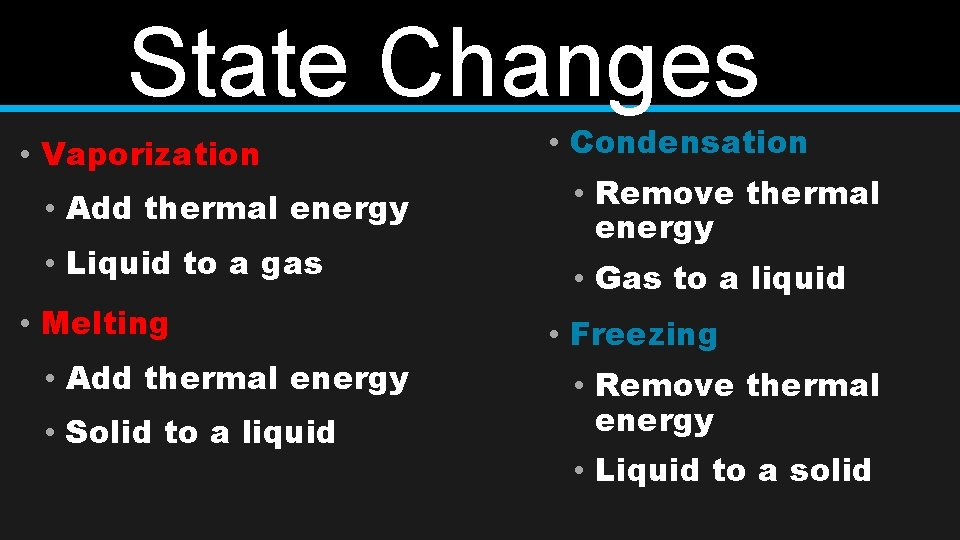State Changes • Vaporization • Add thermal energy • Liquid to a gas • Melting • Add thermal energy • Solid to a liquid • Condensation • Remove thermal energy • Gas to a liquid • Freezing • Remove thermal energy • Liquid to a solid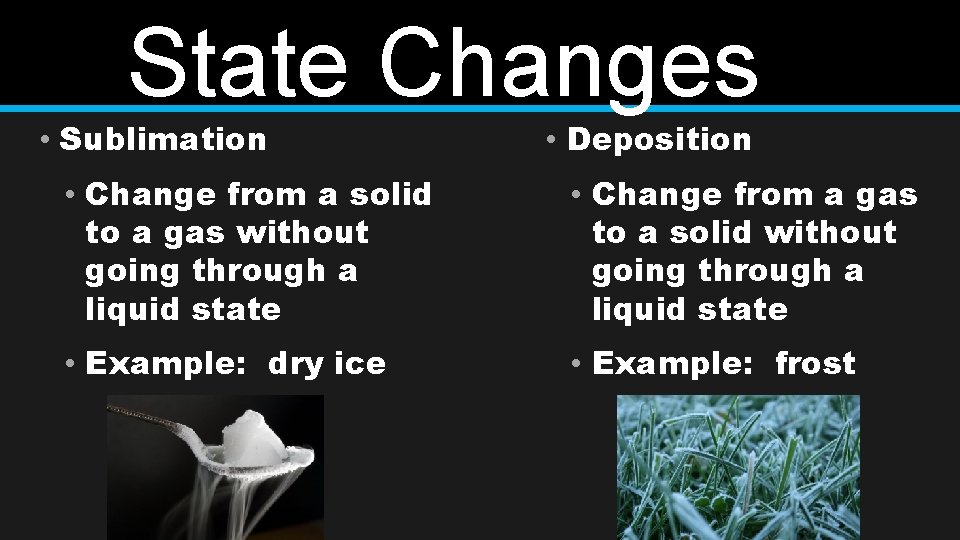State Changes • Sublimation • Deposition • Change from a solid to a gas without going through a liquid state • Change from a gas to a solid without going through a liquid state • Example: dry ice • Example: frost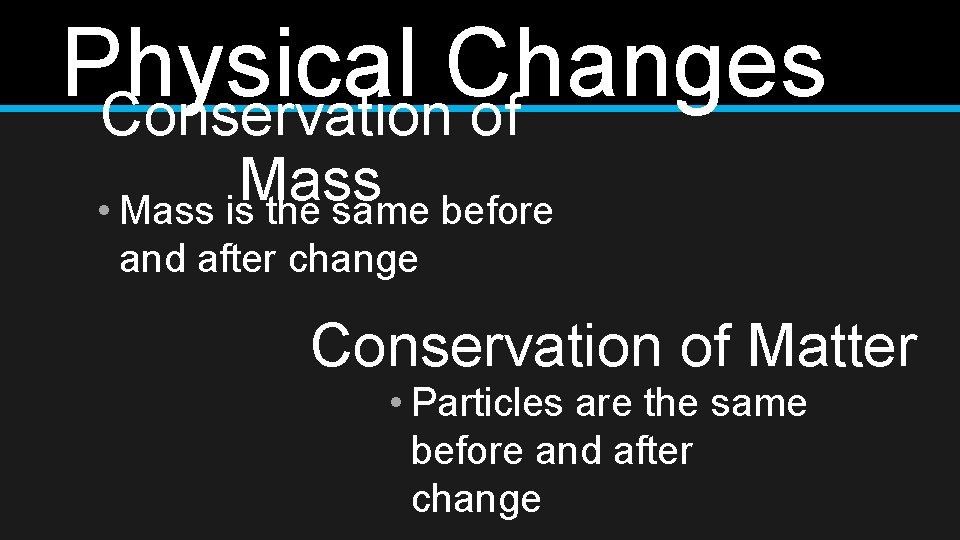Physical Changes Conservation of Mass • Mass is the same before and after change Conservation of Matter • Particles are the same before and after change